# Successive Approximations, Method of

The following article is from The Great Soviet Encyclopedia (1979). It might be outdated or ideologically biased.

## Successive Approximations, Method of

a method of solving mathematical problems by means of a sequence of approximations that converges to the solution and is constructed recursively— that is, each new approximation is calculated on the basis of the preceding approximation; the choice of the initial approximation is, to some extent, arbitrary. The method is used to approximate the roots of algebraic and transcendental equations. It is also used to prove the existence of a solution and to approximate the solutions of differential, integral, and integro-differential equations. Other uses include the obtaining of a qualitative characterization of a solution.

To solve the equation

(1) f(x) = 0

we can form the equivalent equation x = Φ(x), where Φ(x) denotes, for example, the difference xkf(x), where k is a constant. We select an initial approximation a0 to the root of the equation and then construct the sequence of numbers a0, a1 = Φ(a0), a2 = Φ(a1)..... an = Φ(an-1), …. If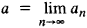exists, it is a root of equation (1); the numbers a0, a1, a2,…, an,.......... are approximations to this root. The limit a exists if, for example,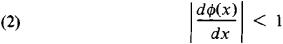and any number may be taken as the initial approximation a0.

Usually when it is necessary to approximate the root of an equation, a sufficiently small interval is established within which the root lies; this may be done, for example, by graphical methods. A number k is then chosen so that condition (2) is satisfied over the interval. Any number in the interval can be selected as the initial approximation a0, whereupon the method of successive approximations is applied. In actual practice, once two successive approximations an — 1 and an differ by less than a specified amount, the computation is halted, and we set a ≈ an.

Suppose, for example, the equation f(x) = ex — l/x = 0 is given. Since f(l/2)f(1) < 0, a root of the equation will lie in the interval (1/2, 1). If we set Φ (x) = x — k(ex — 1 /x), it is easy to see that condition (2) is satisfied over the entire interval (1/2, 1) when k = 1/4. Let us choose a0 = 3/4 and apply the method of successive approximations to the equation x = x — (1/4)- (ex - 1 /x). We obtain a1 = 0.554, a2= 0.570, and a3= 0.566; in fact, the root of the equation, correct to three places, is a ≈ 0.567.

The method of successive approximations is used in the approximate solution of systems of linear algebraic equations with a large number of unknowns. Suppose we are given the system of three equations with three unknowns

a11x + a12y + a13z = b1

(3) a21x + a22y + a23z = b2

a31x + a32y + a33z = b3

We construct the equivalent system

x = c11x + c12y + cl3z + d1

(4) y = c2lx + c22y + c23z + d2

z = c31x + c32y + c33z + d3

setting, for example,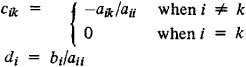where i, k = 1, 2, 3. Using the recurrence formulas

Xj = C11xj –1 + Cl2yj–l + Cl3zj–l + d1

yj = c21xj–1 + C22yj–1 + C23zj–l + d2

zj = C31xj–1 + C23yj–1 + C23zj–l + d3

we form the sequence (x0, y0, Z0),…. (x1, y1, z1), … (xn, yn zn)…. If xn →α, ynβ, and zn → γ as n increases without bound, the three numbers x = a, y = β, and z = γ are the solution of system (3). The limits α, β, and γ clearly exist for arbitrary initial approximations x0 y0 and z0 if, for example, the sum of the absolute values of the coefficients cij in each equation of system (4) is less than unity.

In order to find a solution y = y(x) of the differential equation dy/dx = f(x, y) under the condition y0 = y(x0) we write the equation in the form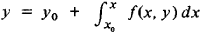Using the recurrence formula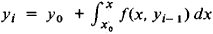we construct the sequence of functions y1(x), y2(x),…., yn(x)…. If this sequence converges uniformly, its limit is the desired solution.

To find the solution of the first boundary value problem for the equation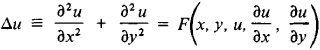an arbitrary, twice differentiable function u0 (x, y) is selected and the linear equation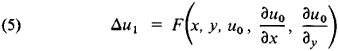then formed. Suppose u1 (x, y) is a solution of the first boundary value problem for equation (5). We can take u1 as the first approximation and then construct equations of the type of equation (5) for the succeeding approximations. The resulting sequence {un (x, y)} converges under certain assumptions and yields a solution of the problem.